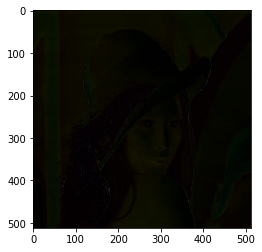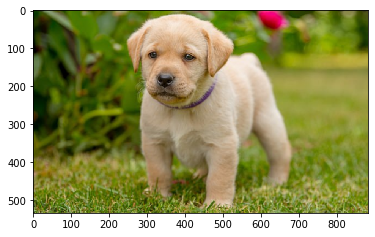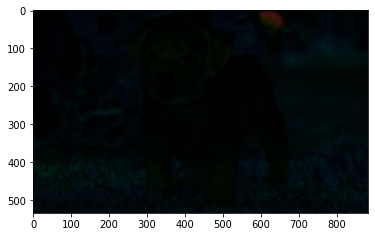Open In App

# Mahotas – Getting Infocusness of each pixel

In this article we will see how we can get the infocusness of each pixel in mahotas. In order to get the infocusness of each pixel we will use the sobel operator. The Sobel operator, sometimes called the Sobel–Feldman operator or Sobel filter, is used in image processing and computer vision, particularly within edge detection algorithms where it creates an image emphasizing edges.

In this tutorial we will use “lena” image, below is the command to load it.

`mahotas.demos.load('lena')`

Below is the lena imageIn order to do this we will use mahotas.sobel method
Syntax : mahotas.sobel(2d_img)
Argument : It takes two dimensional image object as argument
Return : It returns image object

Below is the implementation

## Python3

 `# importing required libraries``import` `mahotas``import` `mahotas.demos``from` `pylab ``import` `gray, imshow, show``import` `numpy as np`` ` `# loading image``img ``=` `mahotas.demos.load(``'lena'``)` `# showing image``print``(``"Image"``)``imshow(img)``show()` `# getting infocusness of each pixel``focus ``=` `np.array([mahotas.sobel(t, just_filter ``=` `True``) ``for` `t ``in` `img])` `# showing focus pixel``print``(``"Focus Image"``)``imshow(focus)``show()`

Output :

`Image``Focus Image`Another example

## Python3

 `# importing required libraries``import` `mahotas``import` `numpy as np``from` `pylab ``import` `gray, imshow, show``import` `os`` ` `# loading image``img ``=` `mahotas.imread(``'dog_image.png'``)``      ` `# filtering image``img ``=` `img[:, :, :``3``]` `# showing image``print``(``"Image"``)``imshow(img)``show()` `# getting infocusness of each pixel``focus ``=` `np.array([mahotas.sobel(t, just_filter ``=` `True``) ``for` `t ``in` `img])` `# showing focus pixel``print``(``"Focus Image"``)``imshow(focus)``show()`

Output :

`Image ``Focus Image`